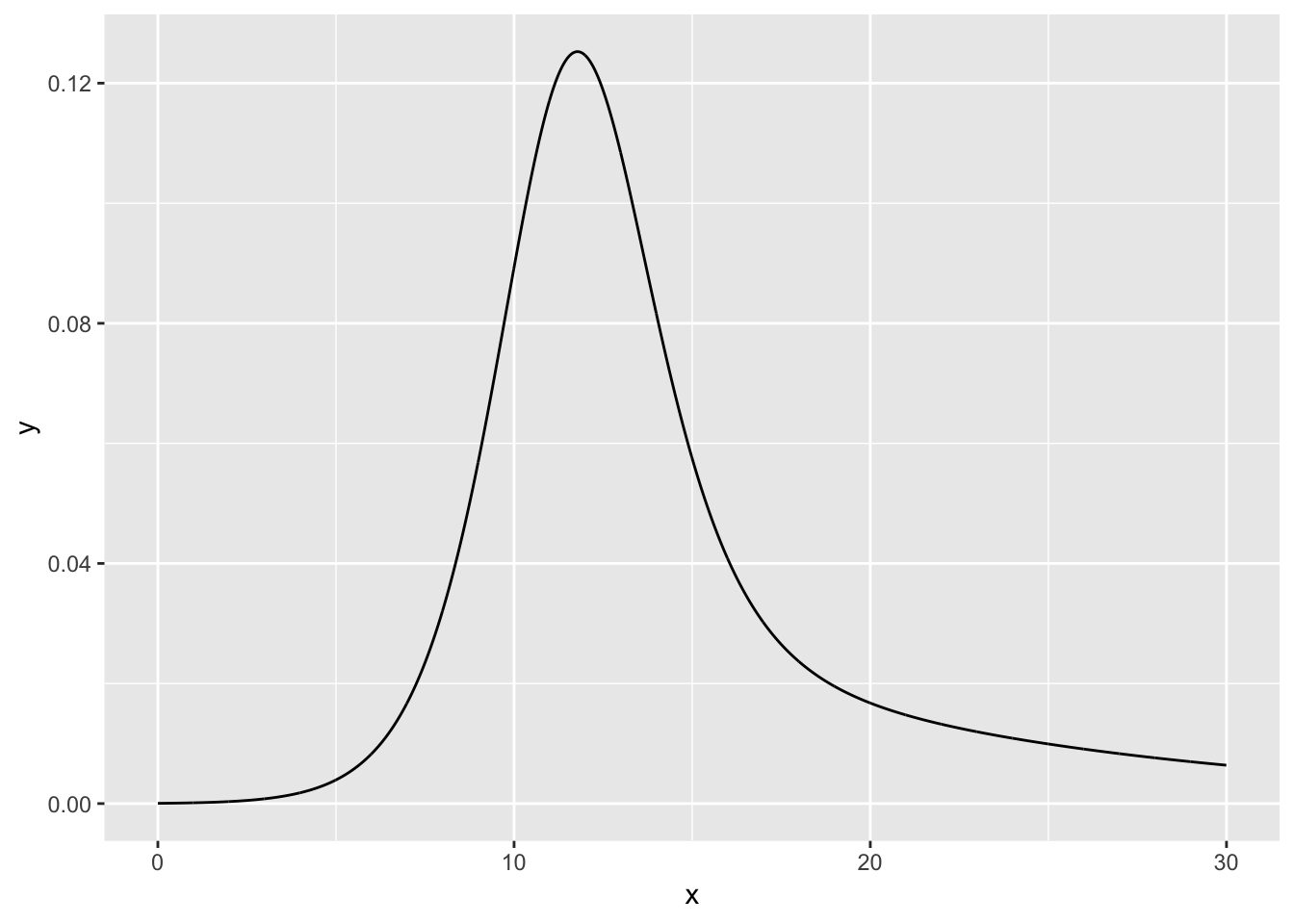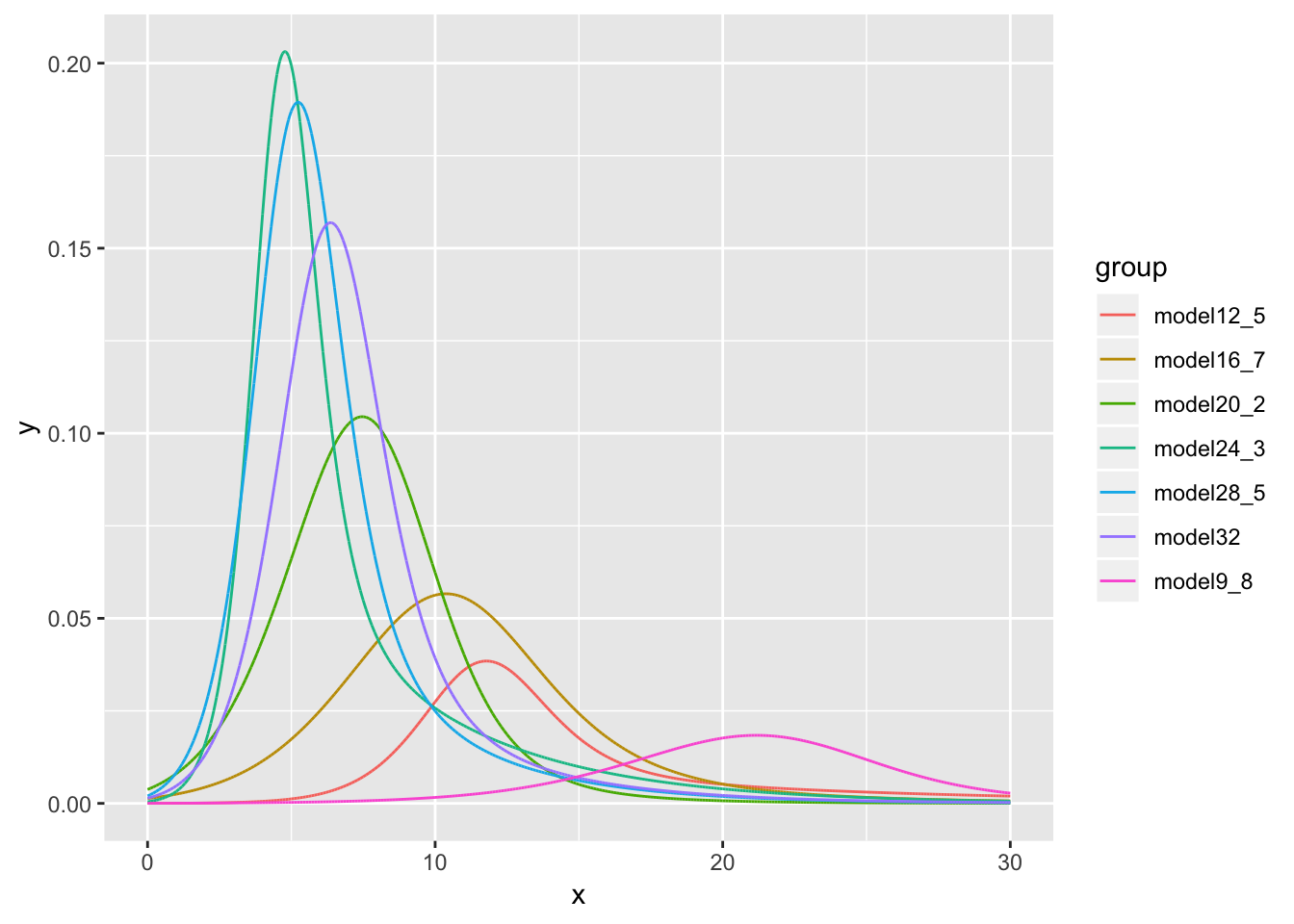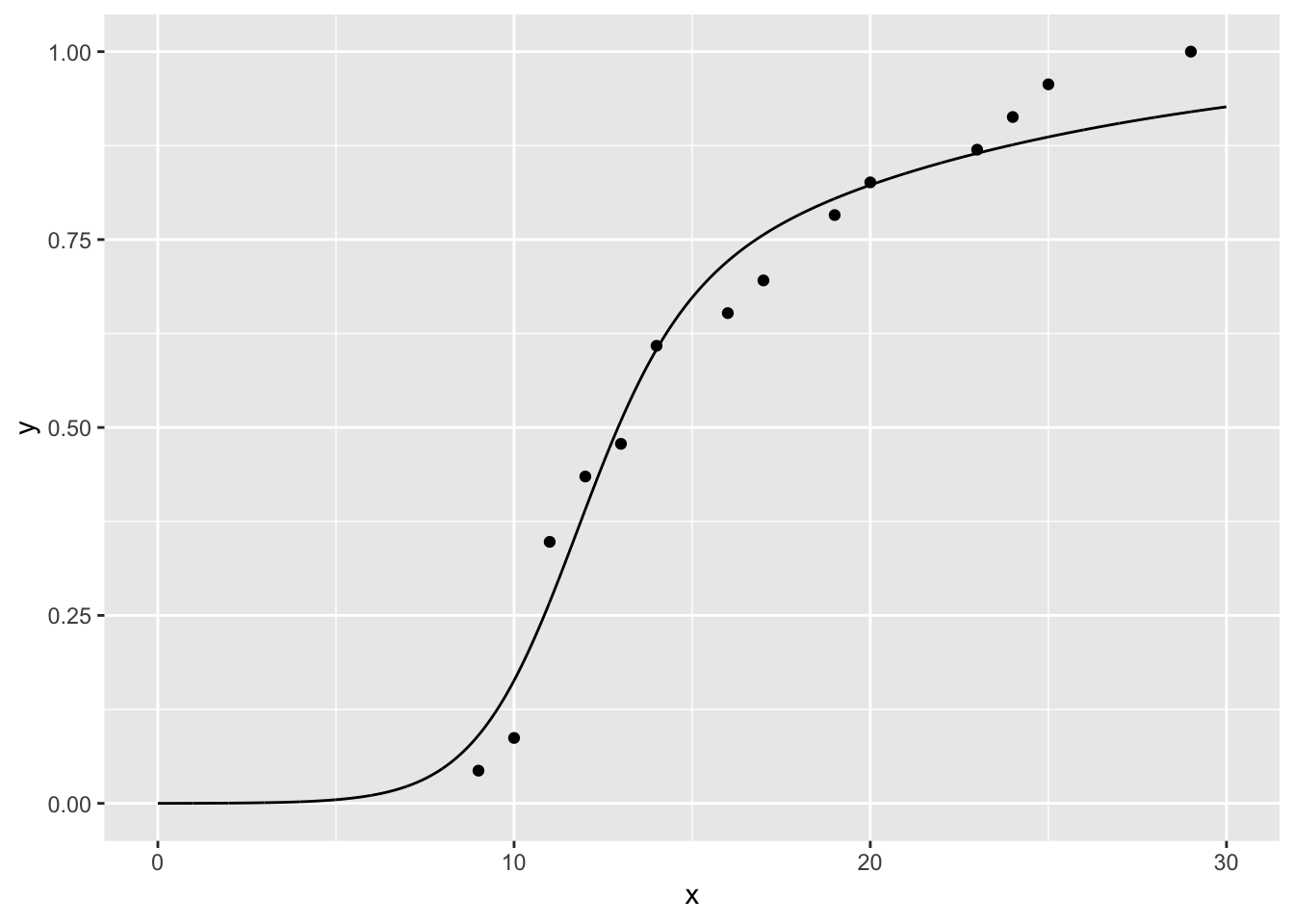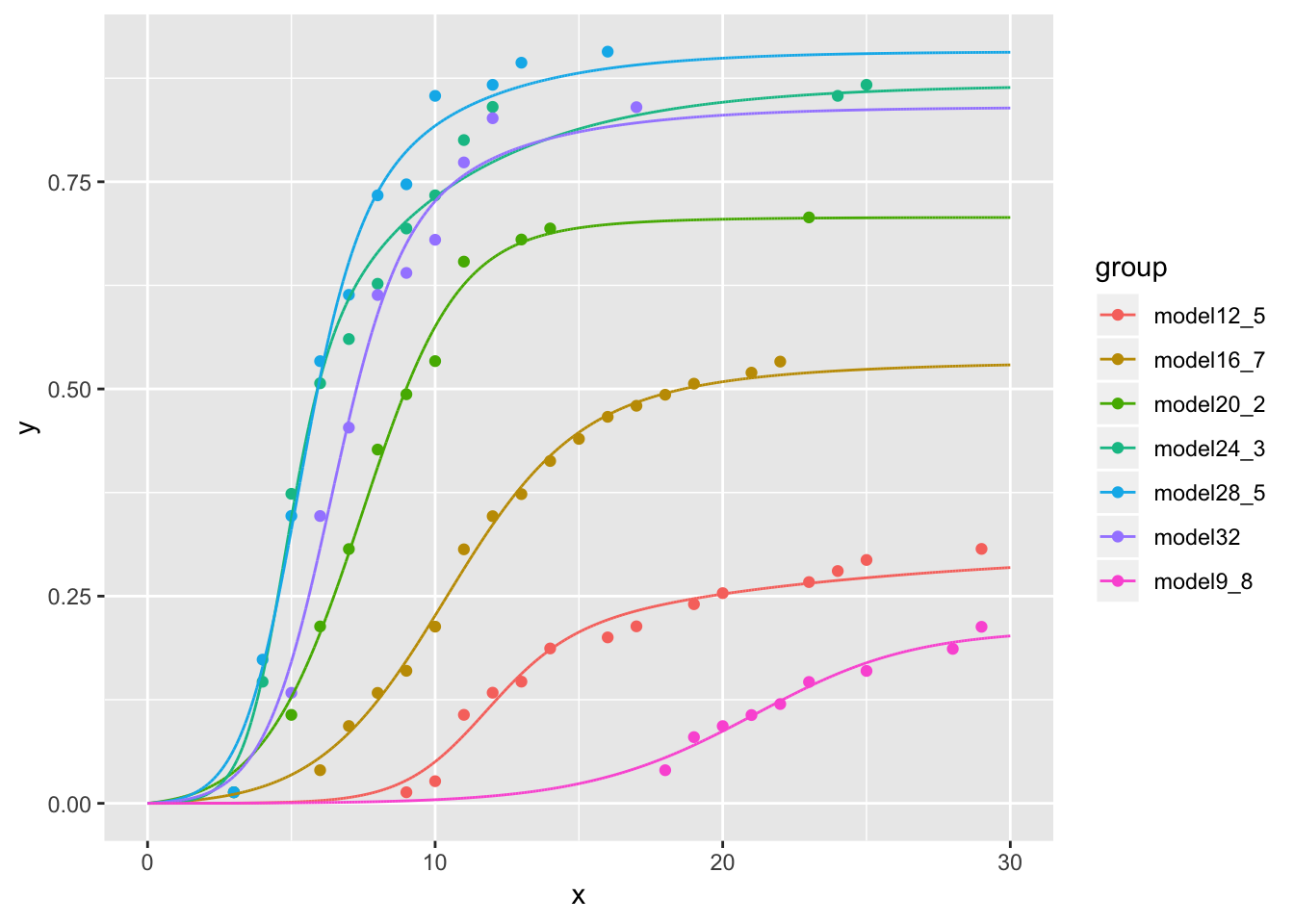# Introduction

This vignette presents the nlstimedist package, a method to fit a new distribution model to the time distribution of a biological phenomenon (Franco 2016). The model differentiates between three essential aspects of a time distribution: the rate at which the process is expected to occur (parameter $$r$$), the rate of change of $$r$$ with time, which is reflected in the time concentration of the distribution (parameter $$c$$), and a measure of the overall distribution time lag (parameter $$t$$). The fitting method incorporates the minpack.lm::nlsLM function (Elzhov et al. 2016) to estimate these three parameters and to plot the estimated time distribution. The nlstimedist package, however, also estimates the standard distribution moments. The method is being proposed to analyse the time distribution of biological events such as germination, phenology, invasion, conclusion of a race, etc. Because parameter values have clear, unique effects on three different aspects of the distribution’s shape (and are correlated but not identical to specific moments), they have clear biological interpretation. This allows the user to further investigate the effect that biological (e.g., species, gender, health, etc.) and environmental factors (e.g., temperature) have on a biological time course. For example, are differences between the sexes in the completion of a marathon race reflected in a particular parameter? If so, what do these differences mean in terms of their size, musculature, aerobic capacity, etc.? If the parameters have a biological interpretation, how are they affected by ambient temperature, hydration, sugar levels, etc.?

# Data Setup

In the model, time is represented by variable $$x$$ and the biological phenomenon is represented by variable $$y$$. The values in each $$y$$ column should be proportions and should be calculated from the cumulative number of events. This must be completed for each column in a dataset. If data have been set up in this manner, skip ahead to the modelling section. If the data have not been set up in this format and it is in a raw format of counts vs. time, they must first be cleaned using the tdData function.

## Cleaning data using tdData

The nlstimedist package comes with several example datasets, one being the lobelia dataset.

head(lobelia)
# A tibble: 6 x 3
Day Temperature Germination
<int>       <dbl>       <int>
1     0         9.8           0
2     1         9.8           0
3     2         9.8           0
4     3         9.8           0
5     4         9.8           0
6     5         9.8           0

We can clean and prepare the data for modelling using the tdData function.

tdLobelia <- tdData(lobelia, x = "Day", y = "Germination",
group = "Temperature")
tdLobelia
# A tibble: 82 x 5
Day Temperature Germination  cumN propMax
<int>       <dbl>       <dbl> <dbl>   <dbl>
1    18         9.8           3     3  0.188
2    19         9.8           3     6  0.375
3    20         9.8           1     7  0.438
4    21         9.8           1     8  0.5
5    22         9.8           1     9  0.562
6    23         9.8           2    11  0.688
7    25         9.8           1    12  0.75
8    28         9.8           2    14  0.875
9    29         9.8           2    16  1
10     9        12.5           1     1  0.0435
# … with 72 more rows

# Modelling the Data

The model is fitted by nonlinear regression employing the Levenberg-Marquardt algorithm. This requires three starting values for $$r$$, $$c$$ and $$t$$, respectively.

## Starting Values

Suggestions for appropriate starting values for each parameter are as follows:

Parameter Recommendation
$$r$$ $$\frac{1}{\text{the period of the time course}}$$, e.g., if completion of the process (all individual events) occurred in 25 days, an appropriate starting value for $$r$$ would be around $$\frac{1}{25}$$ = 0.04.
$$c$$ This requires some trial and error with your particular dataset. We suggest you start with 0.5 and increase (or decrease) it along a logarithmic scale to get a feel of how it is changing. Increasing values of $$c$$ reduce the spread of the distribution: $$c$$ is a measure of concentration of the distribution.
$$t$$ This tends to be close to the mid-point of the monitoring period, but it varies with the skew produced by the combination of parameter values. Nonetheless, as a rule of thumb choose a number near the middle of your time range – if completion of a process (e.g., a marathon race) was closed after 10 hours, choose $$t = 5$$.

## The timedist Function

The model is fitted to the data using the timedist function.

# Fitting the model to data already in the format x = time and y = proportion
# of cumulative number of events.
lobelia12_5 <- tdLobelia$clean[tdLobelia$clean$Temperature == 12.5, ] model12_5 <- timedist(lobelia12_5, x = "Day", y = "propMax", r = 0.03, c = 0.5, t = 14.5) model12_5 Nonlinear regression model model: propMax ~ 1 - (1 - (r/(1 + exp(-c * (Day - t)))))^Day data: data r c t 0.08339 0.62678 12.09364 residual sum-of-squares: 0.03901 Number of iterations to convergence: 16 Achieved convergence tolerance: 1.49e-08 ## Fixing Starting Values On rare occasions the model may fail to converge within 50 iterations. This may occur if a very small dataset is used. It is possible to overcome this issue by fixing or setting upper and lower bounds for one of the starting values. The parameter $$r$$ is the most appropriate parameter to do this with. It is suggested that you calculate the starting value for $$r$$ as in the starting values section and set the upper and lower bounds around this figure (see below). modelFix <- timedist(data = lobelia12_5, x = "Day", y = "propMax", r = 0.03, c = 0.5, t = 14.5, upper = c(0.1, Inf, Inf), lower = c(0.01, -Inf, -Inf)) modelFix Nonlinear regression model model: propMax ~ 1 - (1 - (r/(1 + exp(-c * (Day - t)))))^Day data: data r c t 0.08339 0.62678 12.09364 residual sum-of-squares: 0.03901 Number of iterations to convergence: 16 Achieved convergence tolerance: 1.49e-08 # Interpreting the Fit of the Model To assess how well the model has fit the data, and the reliability of parameter estimates, it is suggested that the standard errors, correlations of the estimates, and confidence intervals are obtained. In each example we have used the model model12_5. ## Standard Errors summary(model12_5, correlation = TRUE, symbolic.cor = FALSE) Formula: propMax ~ 1 - (1 - (r/(1 + exp(-c * (Day - t)))))^Day Parameters: Estimate Std. Error t value Pr(>|t|) r 0.083393 0.006615 12.607 6.99e-08 *** c 0.626782 0.156122 4.015 0.00203 ** t 12.093637 0.462246 26.163 2.95e-11 *** --- Signif. codes: 0 '***' 0.001 '**' 0.01 '*' 0.05 '.' 0.1 ' ' 1 Residual standard error: 0.05955 on 11 degrees of freedom Correlation of Parameter Estimates: r c c -0.49 t 0.72 -0.52 Number of iterations to convergence: 16 Achieved convergence tolerance: 1.49e-08 ## Correlation of Parameter Estimates If a higher level of precision is required, the correlation of parameter estimates can be obtained separately. cpe <- vcov(model12_5) cov2cor(cpe) r c t r 1.0000000 -0.4857904 0.7153296 c -0.4857904 1.0000000 -0.5247587 t 0.7153296 -0.5247587 1.0000000 ## Confidence Intervals To produce accurate confidence intervals for the parameters in a nonlinear regression model fit, we can use the confint2 function. confint2(model12_5) 2.5 % 97.5 % r 0.06883386 0.09795187 c 0.28316063 0.97040290 t 11.07624152 13.11103281 ## R-squared There is no direct R-squared for non-linear regression. However, an R-squared value calculated as $$1-\bigg(\frac{\text{Residual Sum of Squares}}{\text{Corrected Sum of Squares}}\bigg)$$ defines a similar quantity for nonlinear regression, is able to describe the proportion of variance explained by the model, and provides a very good estimate of how well the model fits the data. We can extract this value from our model using the tdRSS function. tdRSS(model12_5)  0.9681957 ## Statistical Moments The following statistical moments for the fitted distribution can be calculated: mean, variance, standard deviation, skew, kurtosis and entropy. model12_5$m$getMoments() mean variance sd skew kurtosis entropy 1 15.75401 83.02729 9.111931 2.897078 12.17524 4.491001 ## Percentiles The percentiles of the distribution can also be calculated. This can be achieved for a single percentile or for a sequence of percentiles. # Extracting a single percentile tdPercentiles(model12_5, n = 0.01) 1% 5.913667 # Extracting a sequence of percentiles from 10% to 90% in steps of 10. tdPercentiles(model12_5, n = seq(0.1, 0.9, 0.1)) 10% 20% 30% 40% 50% 60% 70% 9.159305 10.382816 11.269057 12.073122 12.918504 13.952189 15.516796 80% 90% 18.776037 26.446720  # Plotting the Distribution The package has two built-in graphing functions for plotting the estimated distribution as both a probability density function and a cumulative distribution function. ## Probability Density Function (PDF) The PDF is produced using the function tdPdfPlot. This function takes one or more objects produced by the model, a scaling parameter S and values for the x-axis xVals (which includes a value for smoothing the curve), as arguments to produce the PDF plot. tdPdfPlot(model12_5, S = 1, xVals = seq(0, 30, 0.01))Multiple models can be plotted on the same graph by providing the function with multiple model objects. # Extract the individual data lobelia9_8 <- tdLobelia$clean[tdLobelia$clean$Temperature == 9.8, ]
lobelia16_7 <- tdLobelia$clean[tdLobelia$clean$Temperature == 16.7, ] lobelia20_2 <- tdLobelia$clean[tdLobelia$clean$Temperature == 20.2, ]
lobelia24_3 <- tdLobelia$clean[tdLobelia$clean$Temperature == 24.3, ] lobelia28_5 <- tdLobelia$clean[tdLobelia$clean$Temperature == 28.5, ]
lobelia32 <- tdLobelia$clean[tdLobelia$clean\$Temperature == 32, ]

# Create the models
model9_8 <- timedist(lobelia9_8, x = "Day", y = "propMax",
r = 0.1, c = 0.5, t = 25)
model16_7 <- timedist(lobelia16_7, x = "Day", y = "propMax",
r = 0.1, c = 0.5, t = 10)
model20_2 <- timedist(lobelia20_2, x = "Day", y = "propMax",
r = 0.1, c = 0.5, t = 10)
model24_3 <- timedist(lobelia24_3, x = "Day", y = "propMax",
r = 0.1, c = 1, t = 5)
model28_5 <- timedist(lobelia28_5, x = "Day", y = "propMax",
r = 0.1, c = 1, t = 5)
model32 <- timedist(lobelia32, x = "Day", y = "propMax",
r = 0.1, c = 0.5, t = 5)

# Generate the plot
tdPdfPlot(model9_8, model12_5, model16_7, model20_2, model24_3, model28_5,
model32,
S = c(0.213, 0.307, 0.533, 0.707, 0.867, 0.907, 0.840),
xVals = seq(0, 30, 0.001))## Cumulative Distribution Function (CDF)

The CDF is produced using the function tdCdfPlot. This function takes one or more objects produced by the model, a scaling parameter S and values for the x-axis xVals (which includes a value for smoothing the curve), as arguments to produce the CDF plot.

tdCdfPlot(model12_5, S = 1, xVals = seq(0, 30, 0.01))tdCdfPlot(model9_8, model12_5, model16_7, model20_2, model24_3, model28_5,
model32,
S = c(0.213, 0.307, 0.533, 0.707, 0.867, 0.907, 0.840),
xVals = seq(0, 30, 0.001))